# Chapter 8Appendix: Our Notation

 An adequate notation should be understood by at least two people, one of whom may be the author. Abdus Salam (1950).

We adopt a functional mathematical notation that is close to that used by Spivak in his Calculus on Manifolds . The use of functional notation avoids many of the ambiguities of traditional mathematical notation; the ambiguities of traditional notation that can impede clear reasoning in classical mechanics. Functional notation carefully distinguishes the function from the value of the function when applied to particular arguments. In functional notation mathematical expressions are unambiguous and self-contained.

We adopt a generic arithmetic in which the basic arithmetic operations, such as addition and multiplication, are extended to a wide variety of mathematical types. Thus, for example, the addition operator + can be applied to numbers, tuples of numbers, matrices, functions, etc. Generic arithmetic formalizes the common informal practice used to manipulate mathematical objects.

We often want to manipulate aggregate quantities, such as the collection of all of the rectangular coordinates of a collection of particles, without explicitly manipulating the component parts. Tensor arithmetic provides a traditional way of manipulating aggregate objects: Indices label the parts; conventions, such as the summation convention, are introduced to manipulate the indices. We introduce a tuple arithmetic as an alternative way of manipulating aggregate quantities that usually lets us avoid labeling the parts with indices. Tuple arithmetic is inspired by tensor arithmetic but it is more general: not all of the components of a tuple need to be of the same size or type.

The mathematical notation is in one-to-one correspondence with expressions of the computer language Scheme . Scheme is based on the-calculus  and directly supports the manipulation of functions. We augment Scheme with symbolic, numerical, and generic features to support our applications. For a simple introduction to Scheme, see the Scheme appendix. The correspondence between the mathematical notation and Scheme requires that mathematical expressions be unambiguous and self-contained. Scheme provides immediate feedback in verification of mathematical deductions and facilitates the exploration of the behavior of systems.

#### Functions

The value of the function f, given the argument x, is written f(x). The expression f(x) denotes the value of the function at the given argument; when we wish to denote the function we write just f. Functions may take several arguments. For example, we may have the function that gives the Euclidean distance between two points in the plane given by their rectangular coordinates:In Scheme we can write this as:

(define (d x1 y1 x2 y2)
(sqrt (+ (square (- x2 x1)) (square (- y2 y1)))))

Functions may be composed if the range of one overlaps the domain of the other. The composition of functions is constructed by passing the output of one to the input of the other. We write the composition of two functions using the o operation:A procedure h that computes the cube of the sine of its argument may be defined by composing the procedures cube and sin:

(define h (compose cube sin))

(h 2)
.7518269446689928

which is the same as

(cube (sin 2))
.7518269446689928

Arithmetic is extended to the manipulation of functions: the usual mathematical operations may be applied to functions. Examples are addition and multiplication; we may add or multiply two functions if they take the same kinds of arguments and if their values can be added or multiplied:A procedure g that multiplies the cube of its argument by the sine of its argument is

(define g (* cube sin))

(g 2)
7.274379414605454

(* (cube 2) (sin 2))
7.274379414605454

#### Symbolic values

As in usual mathematical notation, arithmetic is extended to allow the use of symbols that represent unknown or incompletely specified mathematical objects. These symbols are manipulated as if they had values of a known type. By default, a Scheme symbol is assumed to represent a real number. So the expression 'a is a literal Scheme symbol that represents an unspecified real number:

(print-expression
((compose cube sin) 'a))
(expt (sin a) 3)

The procedure print-expression simplifies the expression, removes the type tags, and displays it in a readable form. We can use the simplifier to verify a trigonometric identity:

(print-expression
((- (+ (square sin) (square cos)) 1) 'a))
0

Just as it is useful to be able to manipulate symbolic numbers, it is useful to be able to manipulate symbolic functions. The procedure literal-function makes a procedure that acts as a function having no properties other than its name. By default, a literal function is defined to take one real argument and produce one real value. For example, we may want to work with a function f : R --> R:

(print-expression
((literal-function 'f) 'x))
(f x)

(print-expression
((compose (literal-function 'f) (literal-function 'g)) 'x))
(f (g x))

We can also make literal functions of multiple, possibly structured arguments that return structured values. For example, to denote a literal function named g that takes two real arguments and returns a real value (g : R × R --> R) we may write:

(define g (literal-function 'g (-> (X Real Real) Real)))

(print-expression (g 'x 'y))
(g x y)

We may use such a literal function anywhere that an explicit function of the same type may be used.

There is a whole language for describing the type of a literal function in terms of the number of arguments, the types of the arguments, and the types of the values. Here we describe a function that maps pairs of real numbers to real numbers with the expression (-> (X Real Real) Real). Later we will introduce structured arguments and values and show extensions of literal functions to handle these.

#### Tuples

There are two kinds of tuples: up tuples and down tuples. We write tuples as ordered lists of their components; a tuple is delimited by parentheses if it is an up tuple and by square brackets if it is a down tuple. For example, the up tuple v of velocity components v0, v1, and v2 is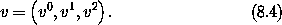The down tuple p of momentum components p0, p1, and p2 isA component of an up tuple is usually identified by a superscript. A component of a down tuple is usually identified by a subscript. We use zero-based indexing when referring to tuple elements. This notation follows the usual convention in tensor arithmetic.

In Scheme we make tuples with the constructors up and down:

(define v (up 'v^0 'v^1 'v^2))

(print-expression v)
(up v^0 v^1 v^2)

(define p (down 'p_0 'p_1 'p_2))

(print-expression p)
(down p_0 p_1 p_2)

Tuple arithmetic is different from the usual tensor arithmetic in that the components of a tuple may also be tuples and different components need not have the same structure. For example, a tuple structure s of phase-space states is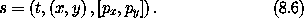It is an up tuple of the time, the coordinates, and the momenta. The time t has no substructure. The coordinates are an up tuple of the coordinate components x and y. The momentum is a down tuple of the momentum components px and py. In Scheme this is written:

(define s (up 't (up 'x 'y) (down 'p_x 'p_y)))

In order to reference components of tuple structures there are selector functions, for example: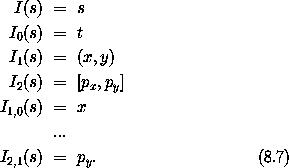The sequence of integer subscripts on the selector describes the access chain to the desired component.

The procedure component is the general selector procedure that implements the selector function Iz:

((component 0 1) (up (up 'a 'b) (up 'c 'd)))
b

To access a component of a tuple we may also use the selector procedure ref, which takes a tuple and an index and returns the indicated element of the tuple:

(ref (up 'a 'b 'c) 1)
b

We use zero-based indexing everywhere. The procedure ref can be used to access any substructure of a tree of tuples:

(ref (up (up 'a 'b) (up 'c 'd)) 0 1)
b

Two up tuples of the same length may be added or subtracted, elementwise, to produce an up tuple, if the components are compatible for addition. Similarly, two down tuples of the same length may be added or subtracted, elementwise, to produce a down tuple, if the components are compatible for addition.

Any tuple may be multiplied by a number by multiplying each component by the number. Numbers may, of course, be multiplied. Tuples that are compatible for addition form a vector space.

For convenience we define the square of a tuple to be the sum of the squares of the components of the tuple. Tuples can be multiplied, as described below, but the square of a tuple is not the product of the tuple with itself.

The meaning of multiplication of tuples depends on the structure of the tuples. Two tuples are compatible for contraction if they are of opposite types, they are of the same length, and corresponding elements have the following property: either they are both tuples and are compatible for contraction, or one of them is not a tuple. If two tuples are compatible for contraction then generic multiplication is interpreted as contraction: the result is the sum of the products of corresponding components of the tuples. For example, p and v introduced in equations (8.4) and (8.5) above are compatible for contraction; the product is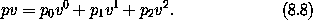So the product of tuples that are compatible for contraction is an inner product. Using the tuples p and v defined above gives us

(print-expression (* p v))
(+ (* p_0 v^0) (* p_1 v^1) (* p_2 v^2))

Contraction of tuples is commutative: pv = vp. Caution: Multiplication of tuples that are compatible for contraction is, in general, not associative. For example, let u = ( 5, 2 ), v = ( 11, 13 ), and g = [ [ 3, 5 ], [ 7, 9 ] ]. Then u (g v) = 964, but (u g) v = 878. The expression u g v is ambiguous. An expression that has this ambiguity does not occur in this book.

The rule for multiplying two structures that are not compatible for contraction is simple. If A and B are not compatible for contraction, the product AB is a tuple of type B whose components are the products of A and the components of B. The same rule is applied recursively in multiplying the components. So if B = ( B0, B1, B2 ), the product of A and B is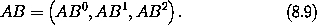If A and C are not compatible for contraction and C = [ C0, C1, C2 ], the product is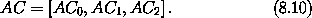Tuple structures can be made to represent linear transformations. For example, the rotation commonly represented by the matrixcan be represented as a tuple structure:1Such a tuple is compatible for contraction with an up tuple that represents a vector. So, for example: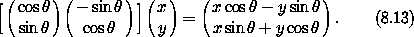Two tuples that represent linear transformations, though not compatible for contraction, may also be combined by multiplication. In this case the product represents the composition of the linear transformations. For example, the product of the tuples representing two rotations isMultiplication of tuples that represent linear transformations is associative but generally not commutative, just as the composition of the transformations is associative but not generally commutative.

#### Derivatives

The derivative of a function f is a function, denoted by Df. Our notational convention is that D is a high-precedence operator. Thus D operates on the adjacent function before any other application occurs: Df(x) is the same as (Df)(x). Higher-order derivatives are described by exponentiating the derivative operator. Thus the nth derivative of a function f is notated as Dn f.

The Scheme procedure for producing the derivative of a function is named D. The derivative of the sin procedure is a procedure that computes cos:

(define derivative-of-sine (D sin))

(print-expression (derivative-of-sine 'x))
(cos x)

The derivative of a function f is the function Df whose value for a particular argument is something that can be multiplied by an incrementx in the argument to get a linear approximation to the increment in the value of f: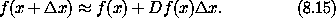For example, let f be the function that cubes its argument (f(x) = x3); then Df is the function that yields three times the square of its argument (Df(y) = 3y2). So f(5) = 125 and Df(5) = 75. The value of f with argument x +x is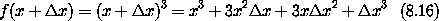andSo Df(x) multiplied byx gives us the term in f(x +x) that is linear inx, providing a good approximation to f(x +x) - f(x) whenx is small.

Derivatives of compositions obey the chain rule: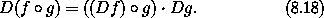So at x,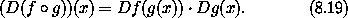Derivatives are operators. An operator is like a function except that multiplication of operators is interpreted as composition, whereas multiplication of functions is multiplication of the values (see equation 8.3). If D were an ordinary function, then the rule for multiplication would imply that D2 f would just be the product of Df with itself, which is not what is intended. Arithmetic is extended to allow manipulation of operators. A typical operator is (D + 1)(D - 1) = D2 - 1, which subtracts a function from its second derivative. The 1 acts as the identity operator: When arithmetically combined with operators, a number is treated as an operator that multiplies its input by the number. Such an operator can be constructed and used in Scheme as follows:

(print-expression
(((* (- D 1) (+ D 1)) (literal-function 'f)) 'x))
(+ (((expt D 2) f) x) (* -1 (f x)))

#### Derivatives of functions of multiple arguments

The derivative generalizes to functions that take multiple arguments. The derivative of a real-valued function of multiple arguments is an object whose contraction with the tuple of increments in the arguments gives a linear approximation to the increment in the function's value.

A function of multiple arguments can be thought of as a function of an up tuple of those arguments. Thus an incremental argument tuple is an up tuple of components, one for each argument position. The derivative of such a function is a down tuple of the partial derivatives of the function with respect to each argument position.

Suppose we have a real-valued function g of two real-valued arguments, and we want to approximate the increment in the value of g from its value at x, y. If the arguments are incremented by the tuple (x,y ) we compute:Using the two-argument literal function g defined above, we have:

(print-expression ((D g) 'x 'y))
(down (((partial 0) g) x y) (((partial 1) g) x y))

In general, partial derivatives are just the components of the derivative of a function that takes multiple arguments (or structured arguments or both; see below). So a partial derivative of a function is a composition of a component selector and the derivative of that function. Indeed:Concretely, if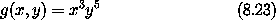then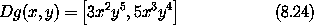and the first-order approximation of the increment for changing the arguments byx andy is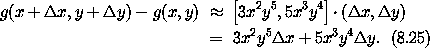Partial derivatives of compositions also obey a chain rule:So if x is a tuple of arguments, then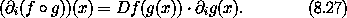Mathematical notation usually does not distinguish functions of multiple arguments and functions of the tuple of arguments. Let h((x, y)) = g(x, y). The function h, which takes a tuple of arguments x and y, is not distinguished from the function g that takes arguments x and y. We use both ways of defining functions of multiple arguments. The derivatives of both kinds of functions are compatible for contraction with a tuple of increments to the arguments. Scheme comes in handy here:

(define (h s)
(g (ref s 0) (ref s 1)))

(print-expression
(h (up 'x 'y)))
(g x y)

(print-expression ((D g) 'x 'y))
(down (((partial 0) g) x y) (((partial 1) g) x y))

(print-expression ((D h) (up 'x 'y)))
(down (((partial 0) g) x y) (((partial 1) g) x y))

A phase-space state function is a function of time, coordinates, and momenta. Let H be such a function. The value of H is H(t, ( x, y ), [ px , py ] ) for time t, coordinates ( x, y ) , and momenta [ px , py ]. Let s be the phase-space state tuple as in (8.6):The value of H for argument tuple s is H(s). We use both ways of writing the value of H.

We often show a function of multiple arguments that include tuples by indicating the boundaries of the argument tuples with semicolons and separating their components with commas. If H is a function of phase-space states with arguments t, ( x, y ), and [ px, py ], we may write H(t; x, y ; px, py) . This notation loses the up/down distinction, but our semicolon-and-comma notation is convenient and reasonably unambiguous.

The derivative of H is a function that produces an object that can be contracted with an increment in the argument structure to produce an increment in the function's value. The derivative is a down tuple of three partial derivatives. The first partial derivative is the partial derivative with respect to the numerical argument. The second partial derivative is a down tuple of partial derivatives with respect to each component of the up-tuple argument. The third partial derivative is an up tuple of partial derivatives with respect to each component of the down-tuple argument: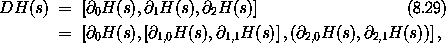where1,0 indicates the partial derivative with respect to the first component (index 0) of the second argument (index 1) of the function, and so on. Indeed,z F = Iz o D F for any function F and access chain z. So, if we lets be an incremental phase-space state tuple,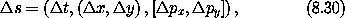thenCaution: Partial derivative operators with respect to different structured arguments generally do not commute.

In Scheme we must make explicit choices. We usually assume phase-space state functions are functions of the tuple. For example,

(define H
(literal-function 'H
(-> (UP Real (UP Real Real) (DOWN Real Real)) Real)))

(print-expression
(H s))
(H (up t (up x y) (down p_x p_y)))

(print-expression
((D H) s))
(down
(((partial 0) H) (up t (up x y) (down p_x p_y)))
(down (((partial 1 0) H) (up t (up x y) (down p_x p_y)))
(((partial 1 1) H) (up t (up x y) (down p_x p_y))))
(up (((partial 2 0) H) (up t (up x y) (down p_x p_y)))
(((partial 2 1) H) (up t (up x y) (down p_x p_y)))))

#### Structured results

Some functions produce structured outputs. A function whose output is a tuple is equivalent to a tuple of component functions each of which produces one component of the output tuple.

For example, a function that takes one numerical argument and produces a structure of outputs may be used to describe a curve through space. The following function describes a helical path around the z-axis in three-dimensional space: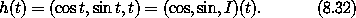The derivative is just the up tuple of the derivatives of each component of the function: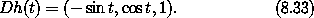In Scheme we can write

(define (helix t)
(up (cos t) (sin t) t))

or just

(define helix (up cos sin identity))

Its derivative is just the up tuple of the derivatives of each component of the function:

(print-expression ((D helix) 't))
(up (* -1 (sin t)) (cos t) 1)

In general, a function that produces structured outputs is just treated as a structure of functions, one for each of the components. The derivative of a function of structured inputs that produces structured outputs is an object that when contracted with an incremental input structure produces a linear approximation to the incremental output. Thus, if we define function g by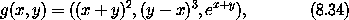then the derivative of g isIn Scheme:

(define (g x y)
(up (square (+ x y)) (cube (- y x)) (exp (+ x y))))

(print-expression ((D g) 'x 'y))
(down (up (+ (* 2 x) (* 2 y))
(+ (* -3 (expt x 2)) (* 6 x y) (* -3 (expt y 2)))
(* (exp y) (exp x)))
(up (+ (* 2 x) (* 2 y))
(+ (* 3 (expt x 2)) (* -6 x y) (* 3 (expt y 2)))
(* (exp y) (exp x))))

Exercise 8.1.  Chain rule
Let F(x, y) = x2 y3, G(x, y) = (F(x, y), y), and H(x, y) = F(F(x, y), y), so that H = F o G.

a.  Compute0 F(x, y) and1 F(x, y).

b.  Compute0 F(F(x, y), y) and1 F(F(x, y), y).

c.  Compute0 G(x, y) and1 G(x, y).

d.  Compute DF(a, b), DG(3, 5) and DH(3a2, 5b3).

Exercise 8.2.  Computing derivatives
We can represent functions of multiple arguments as procedures in several ways, depending upon how we wish to use them. The simplest idea is to identify the procedure arguments with the function's arguments.

For example, we could write implementations of the functions that occur in exercise 8.1 as follows:

(define (f x y)
(* (square x) (cube y)))
(define (g x y)
(up (f x y) y))
(define (h x y)
(f (f x y) y))

With this choice it is awkward to compose a function that takes multiple arguments, such as f, with a function that produces a tuple of those arguments, such as g. Alternatively, we can represent the function arguments as slots of a tuple data structure, and then composition with a function that produces such a data structure is easy. However, this choice requires the procedures to build and take apart structures.

For example, we may define procedures that implement the functions above as follows:

(define (f v)
(let ((x (ref v 0))
(y (ref v 1)))
(* (square x) (cube y))))
(define (g v)
(let ((x (ref v 0))
(y (ref v 1)))
(up (f v) y)))
(define h (compose f g))

Repeat exercise 8.1 using the computer. Explore both implementations of multiple-argument functions.

1 The arrangement of the components of a tuple structure is not significant, as it is in matrix notation: We could just as well have written this tuple as [ ( cos, sin), ( - sin, cos) ].